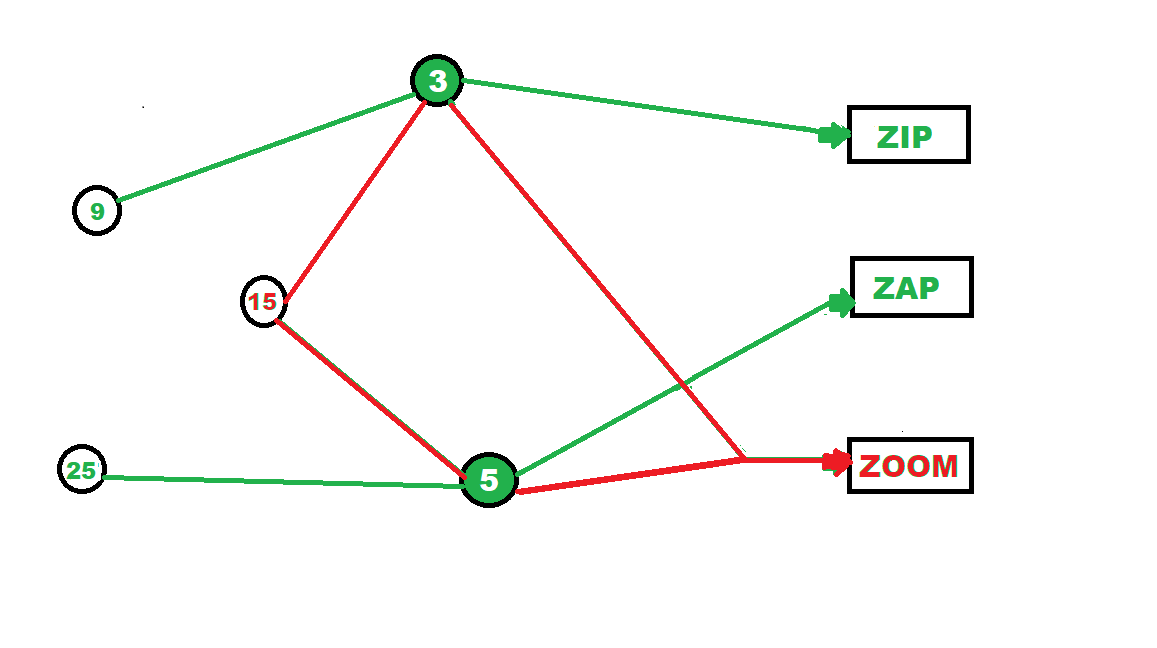# Python program for Zip, Zap and Zoom game

• Last Updated : 04 Feb, 2021

Zip zap zoom is a fun game that requires its players to concentrate and participate actively. When this game is played with people, this is how it is played:

• Players stand in a circle, 6 feet away from each other
• One player starts the game by clap-pointing and saying zap to the person on its left
• This person now does the same to the person to its right and says zap
• Third person so selected now can choose anyone irrespective of the direction by saying zoom
• Now the person so selected starts the game again with zap and the continues in this fashion
• If somebody messes up in between the game is restarted.

In Python programming this game will be achieved by the following approach.

### Approach

• Take in a number as input from the user.
• Check if the number is multiple of both 3 and 5, if it is then print “Zoom”.
• If it is not, then check if the number is a multiple of 3, if it is then print “Zip”.
• If it is a multiple of 5 instead, print “Zap”.
• Else, print a default statement.

Example:

```Input:6
Output:
Zip```

#### Here is a simple and fun illustration describing the same:Program:

## C++

 `#include ``using` `namespace` `std;` `void` `zzz(``int` `number)``{``    ``if` `(number % 3 == 0 && number % 5 == 0)``        ``cout << ``"Zoom\n"``;``    ``else` `if` `(number % 3 == 0)     ``        ``cout << ``"Zip\n"``;``    ``else` `if` `(number % 5 == 0)       ``        ``cout << ``"Zap\n"``;``    ``else``        ``cout << ``"Invalid number!\n"``; ``}` `// Driver code``int` `main()``{``    ` `    ``// Calling function``    ``zzz(7);``    ``zzz(6);``    ``zzz(5);``    ``zzz(30);``    ` `    ``return` `0;``}` `// This code is contributed by svrrrsvr`

## Python3

 `def` `main():``    ``zzz(``7``)``    ``zzz(``6``)``    ``zzz(``5``)``    ``zzz(``30``)`  `def` `zzz(number):``    ``if` `number ``%` `3` `=``=` `0` `and` `number ``%` `5` `=``=` `0``:``        ``print``(``"Zoom"``)``    ``elif` `number ``%` `3` `=``=` `0``:``        ``print``(``"Zip"``)``    ``elif` `number ``%` `5` `=``=` `0``:``        ``print``(``"Zap"``)``    ``else``:``        ``print``(``"Invalid number!"``)`  `main()`

Output:

```Invalid number!
Zip
Zap
Zoom```

My Personal Notes arrow_drop_up